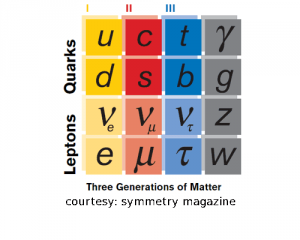# Standard ModelThe standard model of particle physics is a theory concerning the electromagnetic, weak, and strong nuclear interactions, which mediate the dynamics of the known subatomic particles. Developed throughout the early and middle 20th century, the current formulation was finalized in the mid 1970s upon experimental confirmation of the existence of quarks. Since then, discoveries of the bottom quark (1977), the top quark (1995) and the tau neutrino (2000) have given credence to the standard model. Because of its success in explaining a wide variety of experimental results, the standard model is sometimes regarded as a theory of almost everything.

## Lattice Gauge Theory

Lattice gauge theory is the study of gauge theories on a spacetime that has been discretized into a lattice. Gauge theories are important in particle physics, and include the prevailing theories of elementary particles: quantum electrodynamics, quantum chromodynamics (QCD) and the Standard Model. Non-perturbative gauge theory calculations in continuous spacetime formally involve evaluating an infinite-dimensional path integral, which is computationally intractable.

## Effective Field Theory

effective field theory is an approximate theory (usually a quantum field theory) that includes appropriate degrees of freedom to describe physical phenomena occurring at a chosen length scale, while ignoring substructure and degrees of freedom at shorter distances (or, equivalently, at higher energies).

## and QFT foundations

Quantum field theory (QFT) provides a theoretical framework for constructing quantum mechanical models of systems classically parametrized (represented) by an infinite number of dynamical degrees of freedom, that is, fields and (in a condensed matter context) many-body systems. It is the natural and quantitative language of particle physics and condensed matter physics.